# Make way for middle school math!

The top-rated math learning platform for K–5 is now available for Grade 6, too. We’re rolling out new free units for the 2021–22 school year. Join us on October 27th for a live webinar to see the new lessons in action:## What you getDaily digital math lessons where kids independently learn and practice the same concepts they are learning with you, with real-time feedback and built-in math help. Plus, daily lesson plans that help you facilitate learning that builds deep understanding and flexible problem-solving skills. All for free, always.

Daily instructional content covering the first 100 days of the school year are already in all free teacher accounts. We’ll be releasing additional Missions as the 2021–22 school year progresses.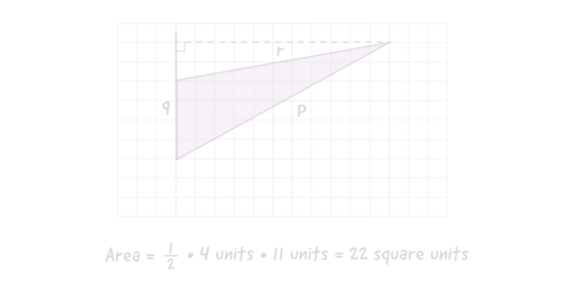### Area and surface area

Students learn to find areas of polygons by decomposing, rearranging, and composing shapes.

Five weeks of instruction
18 digital lessons | 17 lesson plans

M1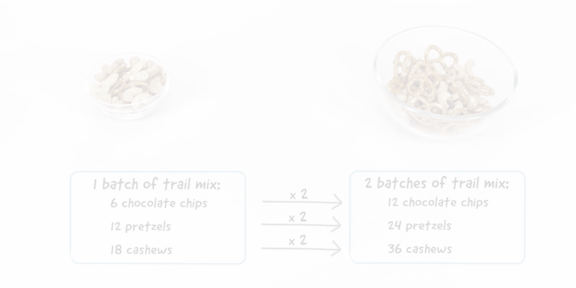### Introducing ratios

Students are introduced to ratios and rates and learn to recognize when two ratios are or are not equivalent.

Four weeks of instruction
16 digital lessons | 17 lesson plans

M2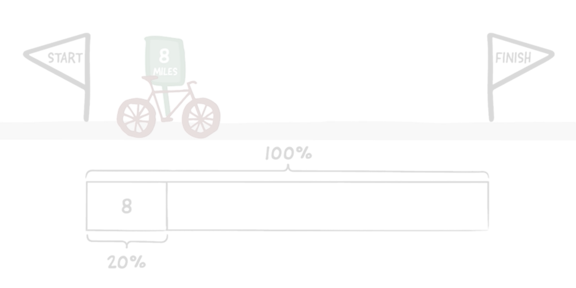### Unit rates and percentages

Students are introduced to unit rate, speed, pace, and percentages and learn to recognize that equivalent ratios have equal unit rates.

Four weeks of instruction
14 digital lessons | 17 lesson plans

M3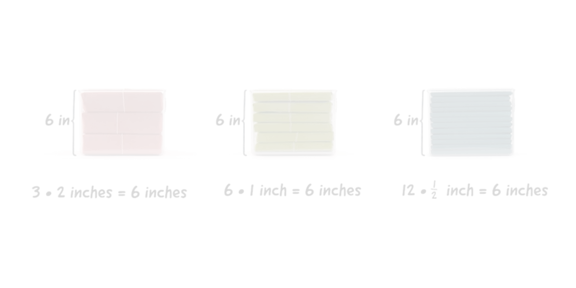### Dividing fractions

Students examine how the relative sizes of numerator and denominator affect the size of their quotient when the numerator or denominator (or both) is a fraction.

Four weeks of instruction
16 digital lessons | 17 lesson plans

M4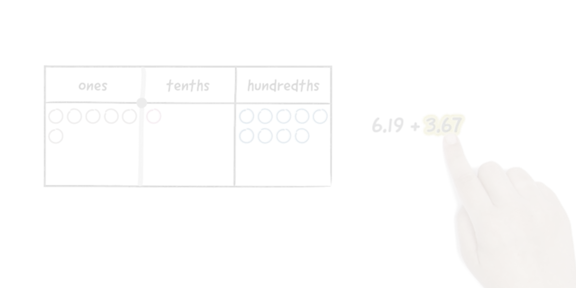### Arithmetic in base ten

Students compute sums, differences, products, and quotients of multi-digit whole numbers and decimals using efficient algorithms.

Four weeks of instruction
14 digital lessons | 15 lesson plans

M5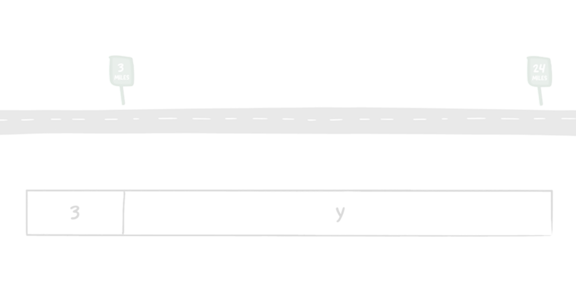### Expressions and equations

Students learn to understand and use the terms “variable,” “coefficient,” “solution,” “equivalent expressions,” “exponent,” “independent variable,” and “dependent variable.”

Four weeks of instruction
16 digital lessons | 18 lesson plans

M6### Coming soon:‍Rational numbers

Students interpret signed numbers in contexts and understand and use the terms “positive number,” “negative number,” “rational number,” “opposite,” “sign,” “absolute value,” “less than,” “greater than,” and the corresponding symbols.

Five weeks of instruction
18 digital lessons | 18 lesson plans

M7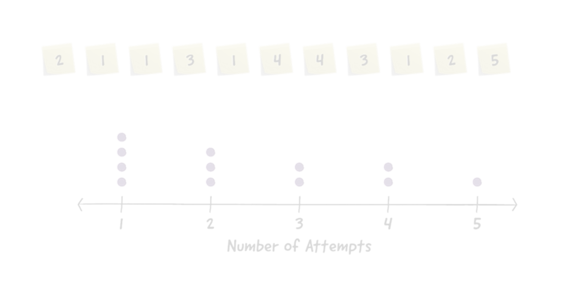### Coming soon:‍Data sets and distributions

Students learn about populations and study variables associated with a population. They understand and use the terms “numerical data,” “categorical data,” “survey,” “statistical question,” “variability,” “distribution,” and “frequency.”

Five weeks of instruction
17 digital lessons | 18 lesson plans

M8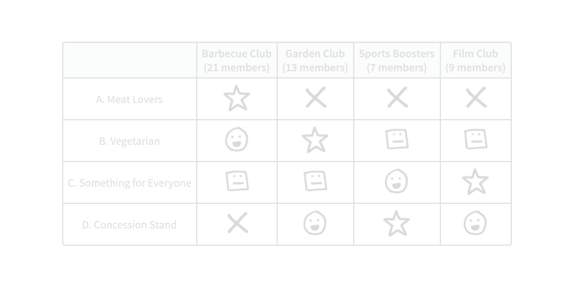### Coming soon:Putting it all together

In this optional Mission, students use concepts and skills from previous Missions to solve Fermi problems, answer questions about ratios of two populations, explore the relationship between the greatest common factor of two numbers, and explore the mathematics of voting.

One week of instruction
6 lesson plans

M9@Zearned just released more units of Grade 6! 100 instructional days of FREE digital lessons covering the same content you’re teaching, with just-in-time support from prior grades & units. Check them out!

## FAQs

### More Zearn Math for Middle School is on the way.